# 机器学习|朴素贝叶斯算法（三）-深入理解朴素贝叶斯原理

+关注继续查看

$$P\left ( H|D \right )=\frac{P\left(H \right )P\left(D|H\right)}{P\left(D\right)}$$

$H$---女生，$D$---穿长裤

$P(H)$称为先验概率，即在得到新数据前某一假设的概率
$P(H|D)$称为后验概率，即在看到新数据后，我们要计算的该假设的概率
$P(D|H)$是该假设下得到这一数据的概率，称为似然
$P(D)$是在任何假设下得到这一数据的概率，称为标准化常量


**

$$P\left(A\right)=P\left(A|B_{1}\right)P\left(B_{1}\right)+P\left(A|B_{2}\right)P\left(B_{2}\right)+$$

$$...+P\left(A|B_{n}\right)P\left(B_{n}\right).$$

$$P\left(B_{i}|A \right )=\frac{P\left(A|B_{i}\right)P\left(B_{i} \right )}{P\left(A \right )}$$

$$=\frac{P\left(A|B_{i} \right )P\left(B_{i} \right )}{\sum_{j=1}^{n}P\left(A|B_{j} \right )P\left(B_{j} \right )},i=1,2,...,n.$$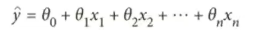【机器学习】回归的原理学习与葡萄酒数据集的最小二乘法算法线性回归实例
【机器学习】回归的原理学习与葡萄酒数据集的最小二乘法算法线性回归实例
0 0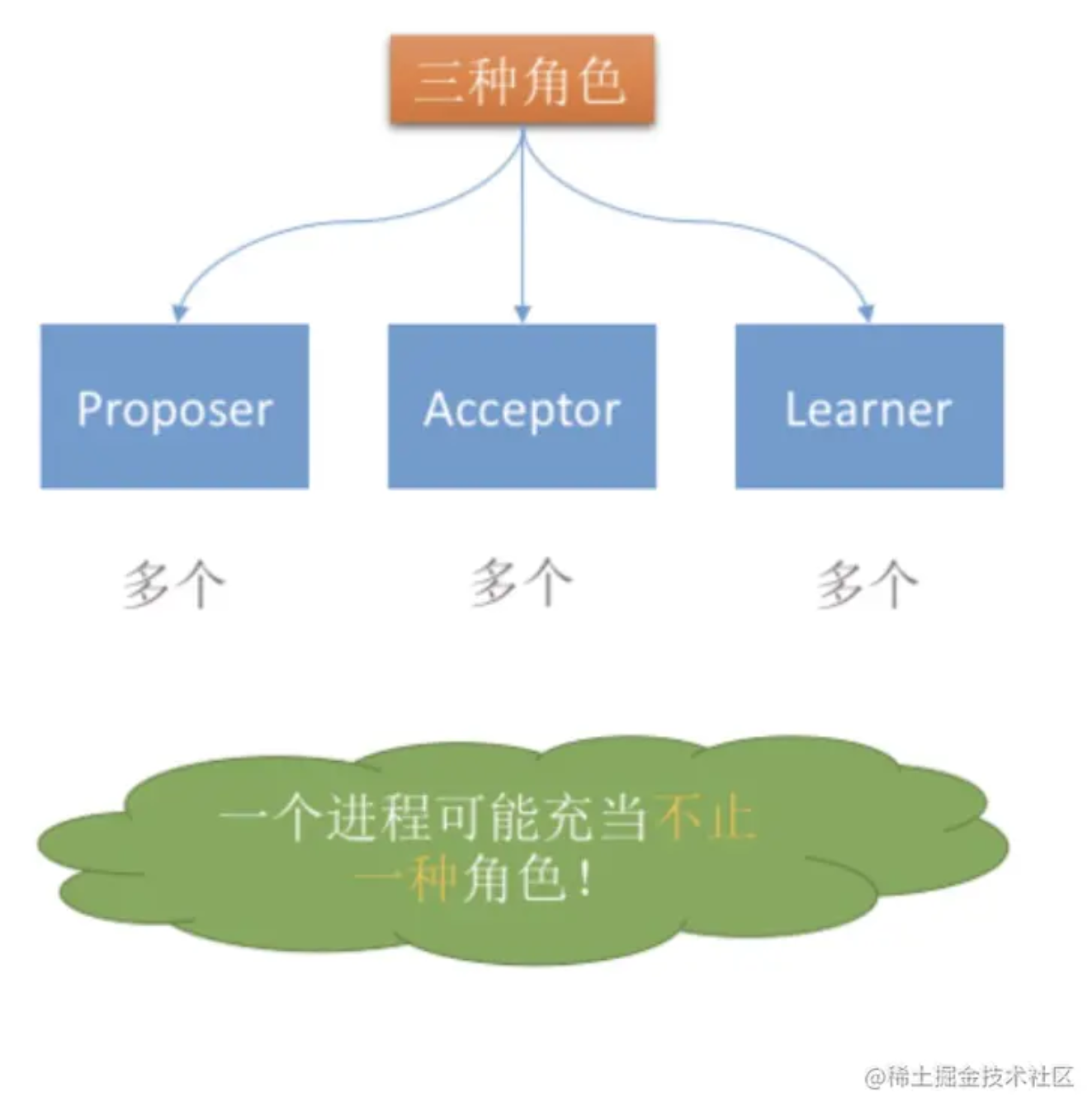【架构师指南】带你彻底认识 Paxos 算法、Zab 协议和 Raft 协议的原理和本质
【架构师指南】带你彻底认识 Paxos 算法、Zab 协议和 Raft 协议的原理和本质
0 0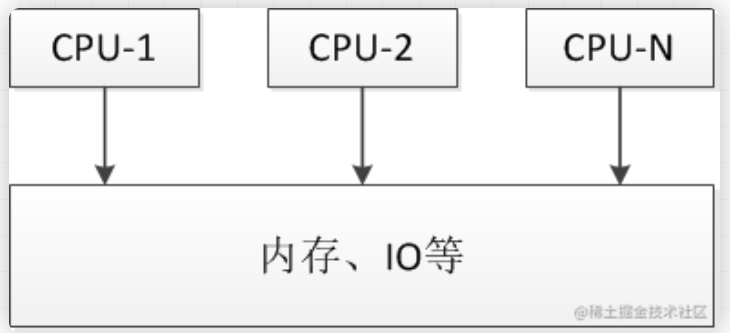【数据结构之旅】「线程锁算法专项」引领你走进CLH队列锁机制原理世界
【数据结构之旅】「线程锁算法专项」引领你走进CLH队列锁机制原理世界
0 0【演化(进化)算法】遗传算法原理及python实现
0 0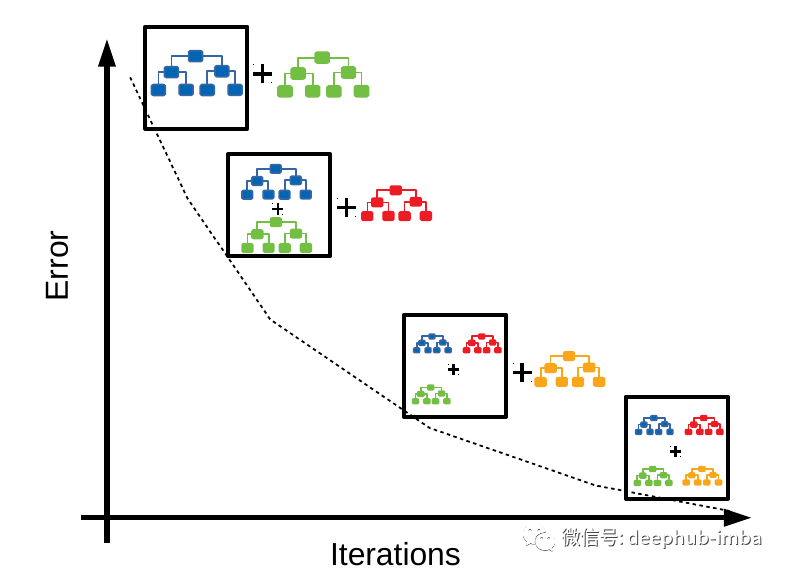0 0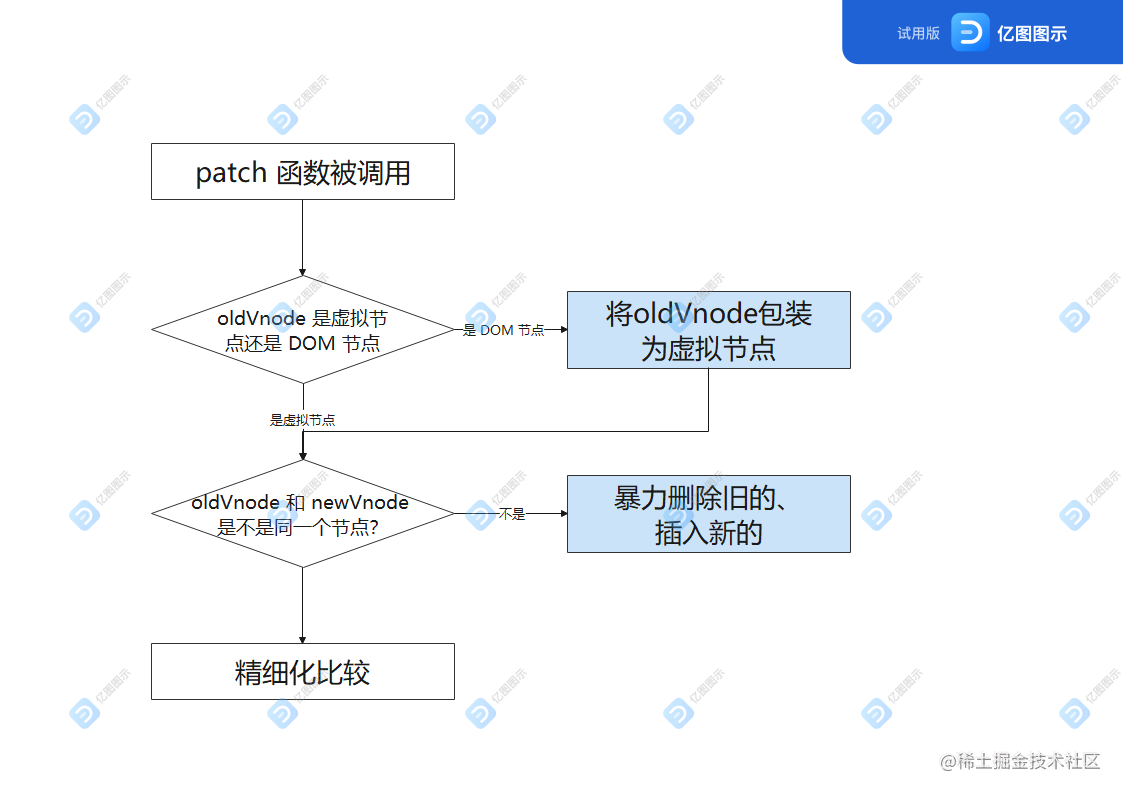0 0
kissjz
Keep It Simple , Stupid. 独立博客：白水东城（www.baishuidongcheng.com）

【算法实战】6. 支持向量机算法4910

【算法实战】4. 朴素贝叶斯算法976

【算法实战】5. Logistic回归算法376

【算法实战】9. 线性回归算法275517

【算法实战】2. K近邻算法1255